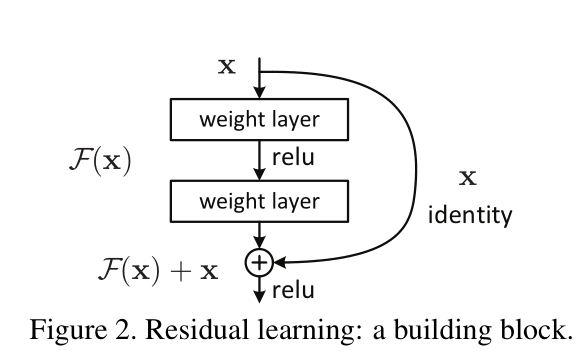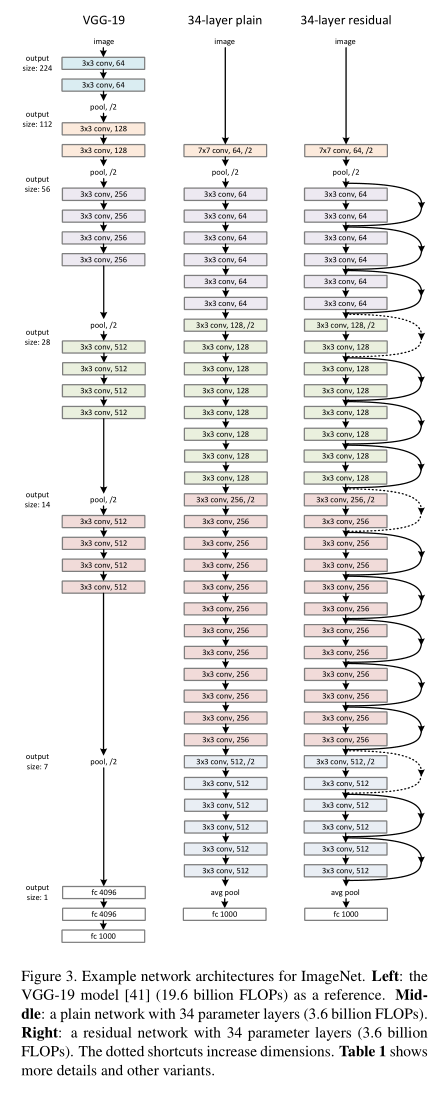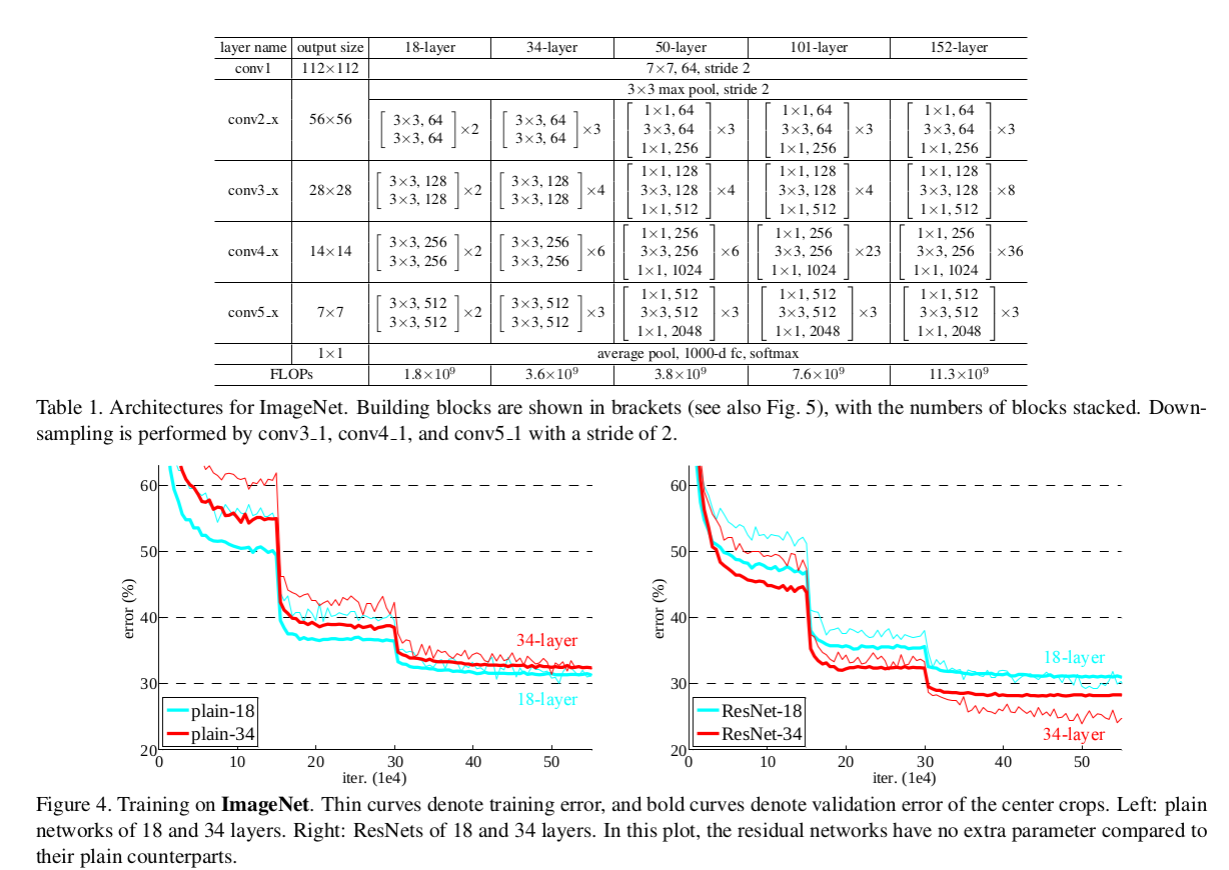## Thursday, October 24, 2019

### Model Distillation

Model Distillation is the process of taking a big model or ensemble of models and producing a smaller model that captures most of the performance of the original bigger model. It could be also better be described as a blind model replication method.

The reasons for doing so are:
1. improved run-time performance (FLOP operations)
2. (maybe) better generalization because of the model simplicity
3. you don't have access to the training of the original model.
4. you have access to a remotely deployed model and you want to replicate it (it happens more than you can imagine)
5. original model maybe is too complicated
6. insights that may arise from the process itself

### How it works

Assume a MNIST classifier $$F_{MNIST}$$ composed of an ensemble of $$N$$ convolutional deep neural networks that produces a logit $$z_i$$ which is then converted to a probability of an input image, $$x_i$$, for each of the possible labels $$C_{0}-C_{9}$$.

The distillation process will give us an  $$F_{MNIST_{distilled}}$$ composed of a single deep neural network that will approximate the classification results of the bigger ensemble of models.

In distillation, knowledge is transferred from the teacher model to the student by minimizing a loss function in which the target is the distribution of class probabilities predicted by the teacher model. That is - the output of a softmax function on the teacher model's logits.

Logits $$z_j$$ are converted to probabilities $$P(C_i|x)$$ using the softmax layer:

$$p_i = \frac {exp(z_i)} {\sum_{j}exp(z_j)}$$

However, in many cases, this probability distribution has the correct class at a very high probability, with all other class probabilities very close to 0. As such, it doesn't provide much information beyond the ground truth labels already provided in the dataset.

To tackle this issue, Hinton et al., 2015 introduced the concept of "softmax temperature". The probability $$q_i$$ is computer by the logit $$z_i$$ for the scalar softmax temperature $$T$$:

$$q_i = \frac {exp(\frac{z_i}{T})} {\sum_{j}exp(\frac{z_j}{T})}$$

where T is a temperature that is normally set to 1. Using a higher value for T produces a softer probability distribution over classes. Softer probability distribution means that the values are somewhat diffused and a 0.999 probability may become 0.9 and the rest spread to the other classes.

In the simplest form of distillation, knowledge is transferred to the distilled model by training it on a transfer set and using a soft target distribution for each case in the transfer set that is produced by using the cumbersome model with a high temperature in its softmax. The same high temperature is
used when training the distilled model, but after it has been trained it uses a temperature of 1. When the correct labels are known for all or some of the transfer set, this method can be significantly improved by also training the distilled model to produce the correct labels. One way to do this is to use the correct labels to modify the soft targets, but we found that a better way is to simply use a weighted average of two different objective functions.
1. The first objective function is the cross entropy with the soft targets and this cross entropy is computed using the same high temperature in the softmax of the distilled model as was used for generating the soft targets from the cumbersome model.
2. The second objective function is the cross entropy with the correct labels. This is computed using exactly the same logits in softmax of the distilled model but at a temperature of 1.
This very simple operation can have a multitude of knobs and parameters to adjust but the core essence is very simple and works quite well.

## Friday, October 18, 2019

### ResNets inner workings and notes

A residual network (or ResNet) is a standard deep neural net architecture, with state-of-the-art performance across numerous applications. The main premise of ResNets is that they allow the training of each layer to focus on fitting just the residual of the previous layer’s output and the target output. Thus, we should expect that the trained network is no worse than what we can obtain if we remove the residual layers and train a shallower network instead.

Up until 2015 we had 3 mainstream ways of training deep networks:
• Greedy per layer optimization and freezing
• Various flavors of Dropout
• and Batch Normalization (came out early 2015, wasn't mainstream until 2016, now it is patented by google so who knows if people can use it)
The main contribution of the "Deep Residual Learning for Image Recognition, 2015" paper is a novel  and smart building block for training very deep neural networks.

### The "Residual Learning" or "Identity Learning" block.A special note here:
We need at least 2 weight layers here (nonlinearities are not essential but welcome) to get the benefits of the universal function approximator that has been proven since the 90's. You can add more but you then hit the limits of information propagation and you will need residual/skip connections inside the residual block.

A stack of $$n$$ residual blocks is described as follows :

$$x_0$$ : input
$$x_1 = x_0 + F_1(x_0)$$
$$x_2 = x_1 + F_2(x_1)$$
$$x_3 = x_2 + F_3(x_2)$$
$$...$$
$$x_n = x_{n-1} + F_{n-1}(x_{n-1})$$

This can be re-written as  :

$$x_n = x_{n-1} + F_{n-1}( x_{n-2} + F_{n-2}(x_{n-2})$$

Which can be expanded as :

$$x_n = x_{n-1} + F_{n-1}( x_{n-2} + F_{n-2}( x_{n-3} +F_{n-3}( x_{n-4} + F_{n-4}(... + F_1(x_0))))))$$

Now if we assume that $$F_i$$ is a linear function (it is not for $$x \lt 0$$ ) but we can ignore it) :

$$x_n = x_{n-1} + F_{n-1}( x_{n-2} ) + F_{n-1}F_{n-2}(x_{n-3}) + F_{n-1}F_{n-2}F_{n-3}(x_{n-4}) + ... F_{n-1}F_{n-2}F_{n-3}...F_1(x_0)$$

As we can see from the equations and the equivalent graph, there is a clear information flow from the raw data to the output. This means that the major pain point of vanishing gradient is avoided.This design when stacked as above allows the gradient to flow through the whole network bypassing any tricky points and in essence training the deeper network properly. Information bottlenecks can be introduced by design but the gradient can flow from the loss layer up to the base layer.

Residual Networks (ResNets) have been used to train up to a 1000 layers. It was proven empirically that going deeper generalizes better than shallow wide networks. Again empirically several variations of the Residual Block are tried:In practice the Residual Function is not 2 (convs or fully connected) weight layers but they are accompanied by a Batch Normalization layer. This helps stabilize the gradient and the co-variate shift (there are various opposing hypothesis on why batch norm works).

In the paper "Identity Mappings in Deep Residual Networks, 2016" a different layout was proposed that improved the performance. We can see an inversion of the order of the layers. The architecture, the analysis and the system design remains the same though.
This new arrangements produces better results on very deep networks for the CIFAR dataset, shown below. Also the convergence is much faster. Since they are practically the same from an engineering perspective we don't have a reason to reject the one with the better performance.